4WX Internet Search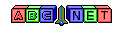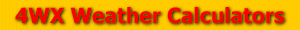# Pressure Altitude

From the user, a station pressure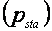is given. In order to calculate pressure altitude, the units for station pressure must be converted to millibars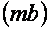or hectopascals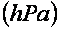For information on how to convert to millibars, see the link below:

Then, the pressure altitude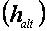can be calculated using the equation below:The answer will be units of feet. To convert the answer to units of meters see the equation below: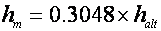Back to 4WX Weather Calculators index page

4WX Calculators Credit and disclaimer